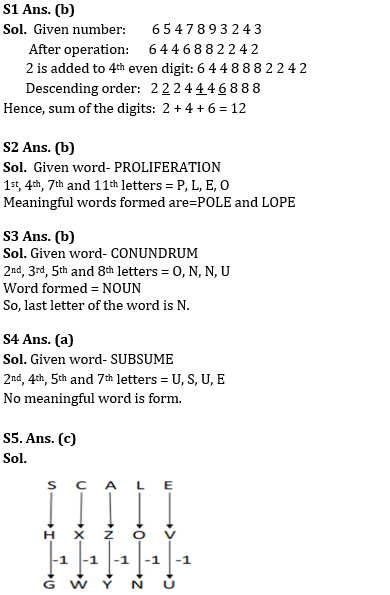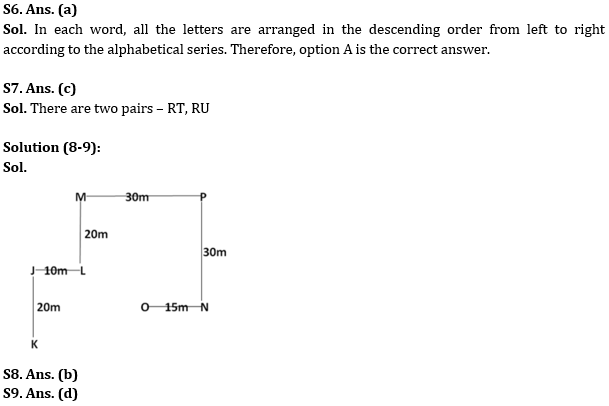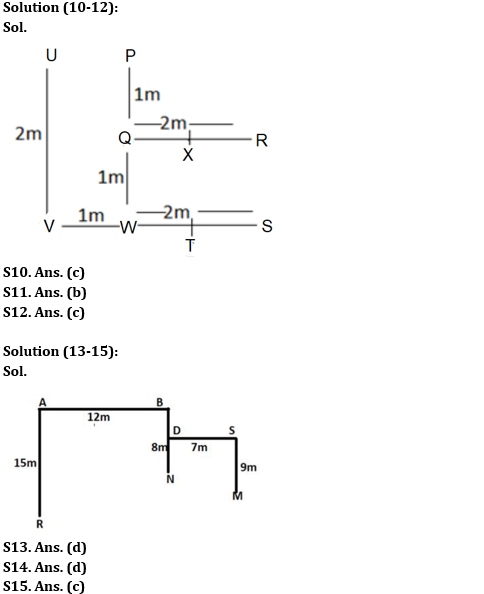Latest Banking jobs   »

# Reasoning Ability Quiz For SBI Clerk Prelims 2022- 23rd May

Q1. In the given number ‘6547893243’, all the odd digits are subtracted by 1 and thus a new number is form. Now, 2 is added to the 4th even digit within the number thus form from the left, then arrange the resultant digit in descending order from right to left. Find the sum of 2nd, 5th and 7th digit from the left end?
(a) 15
(b) 12
(c) 10
(d) 13
(e) 14

Reasoning Ability Quiz For SBI Clerk Prelims 2022- 23rd May CHECK IN HINDI

Q2. If it is possible to make a four-letter meaningful word from the 1st, 4th, 7th and 12th letters from the left end of the word ‘PROLIFERATION’, which of the following will be the third letter of that word? If more than one such word can be formed, then give ‘M’ as the answer and if no such word can be formed, then give ‘X’ as the answer.
(a) X
(b) M
(c) L
(d) E
(e) P

Q3. If it is possible to make a four-letter meaningful word from the 2nd, 3rd, 5th and 8th letters from the left end of the word ‘CONUNDRUM’, which of the following will be the last letter of that word? If more than one such word can be formed, then give ‘Y’ as the answer and if no such word can be formed, then give ‘X’ as the answer.
(a) X
(b) N
(c) Y
(d) U
(e) O

Q4. If it is possible to make a four-letter meaningful word from the 2nd, 4th, 5th and 7th letters from the left end of the word ‘SUBSUME’, which of the following will be the third letter of that word? If more than one such word can be formed, then give ‘M’ as the answer and if no such word can be formed, then give ‘Y’ as the answer.
(a) Y
(b) M
(c) S
(d) E
(e) U

Q5. In a certain code language, if ‘MATCH’ is coded as ‘MYFWR’ and ‘EXTRA’ is coded as ‘UBFHY’, then what is the code for ‘SCALE’?
(a) GWYUN
(b) GWNYU
(c) GWYNU
(d) GWYMU
(e) None of these

Q6. In a certain code language, if ‘DEPLOYING’ is coded as ‘YPONLIGED’ and ‘OFFENSIVE’ is coded as ‘VSONIFFEE’, then what is the code for ‘LENDING’?
(a) NNLIGED
(b) NNLIEGD
(c) NNILGED
(d) NNLIGDE
(e) None of these

Q7. How many such pairs of letters are there in the word ‘QUADRATE’, each of which has as many letters between them in the word as in the English alphabet (Both forward and backward)?
(a) None
(b) One
(c) Two
(d) Three
(e) More than three

Directions (8-9): Study the following information carefully and answer the following questions.
Point N is 15m east of point O. Point P is 30m east of point M. Point J is 20m north of point K and 10m west of point L. Point N is 30m south of point P. Point L is 20m south of point M.

Q8. Point L is in which direction of point O?
(a) South
(b) North-west
(c) South-West
(d) North-East
(e) None of these

Q9. Point P is in which direction of point J?
(a) North
(b) East
(c) South-West
(d) North-East
(e) Can’t be determined

Directions (10-12): Study the following information carefully and answer the following questions.
P, Q, R, S, T, U, V, W and X are nine houses. R is 2m east of Q, which is 1m south of P, which is 2m north of W. V is 1m west of W while S is 3m east of V, which is 2m south of U. X is just in the middle of Q and R while T is just in the middle of W and S.

Q10. How far and in which direction is house U with respect to house P?
(a) 1m, East
(b) 2m, East
(c) 1m, West
(d) 2m, West
(e) 2m, North

Q11. House S is in which direction with respect to house R?
(a) North
(b) South
(c) South-East
(d) Can’t be determined
(e) None of these

Q12. Four of the following five are alike in a certain way and hence form a group. Which of the following pair does not belong to the group?
(a) QW
(b) VW
(c) QR
(d) TS
(e) PQ

Directions (13-15): Study the following information carefully and answer the following questions.
Point B is 8m to the north of Point N. Point A is 12m to the west of Point B. Point R is 15m to the south of Point A. Point S is 7m to the east of Point D which is mid-point of Point B and Point N. Point M is 9m to the south of Point S.

Q13. What is the total distance between Point D and Point M?
(a) 11m
(b) 9m
(c) 10m
(d) 16m
(e) None of these

Q14. What is the total distance between Point R and Point S?
(a) 42m
(b) 31m
(c) 39m
(d) 38m
(e) None of these

Q15. What is the direction of Point A with respect to Point M?
(a) South-east
(b) North-east
(c) North-west
(d) North
(e) None of these

Solutions#### Congratulations!